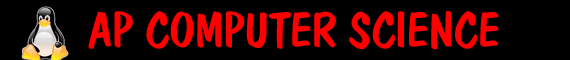Quiz 13a - references   id:   1. If a method takes in primitive data, then changing it in the method will change it where it was called. true false   2. What is true about using == to compare Strings?   it always works, but its better practice to use .equals it works sometimes, but its better practice to use .equals it is fine to use it will give a compile time error it will give a run time error   3. When will this return true: student1==student2 it never is true student1 points to the same object that student2 does. student1 is the same as student2 (same values stored as global variables). compile time error, you can assign equality for objects: you need to use .equals 4. What would the following code print out: ```public void update (int x) { x++; System.out.print(x); } int y=10; update(y); System.out.print(y); ``` 5. What would the following code print out: ```public void update (EasyInt x) { x.setValue(8); System.out.print(x); } EasyInt y=new EasyInt(4); update(y); System.out.print(y); ```   6. EC remember x.toString gives a String that is just the value. if x is an easyInt with value 12, x.toString() would give "12". What would the following code print out: ```EasyInt x=new EasyInt(4); EasyInt y=new EasyInt(5); x=y; x.setValue(9); System.out.print(x.toString()+y.toString()); ``` 7. What would the following code print out: ```EasyInt x=new EasyInt(4); EasyInt y=new EasyInt(5); x=y; x.setValue(9); x=new EasyInt(1); System.out.print(x.toString()+y.toString()); ```